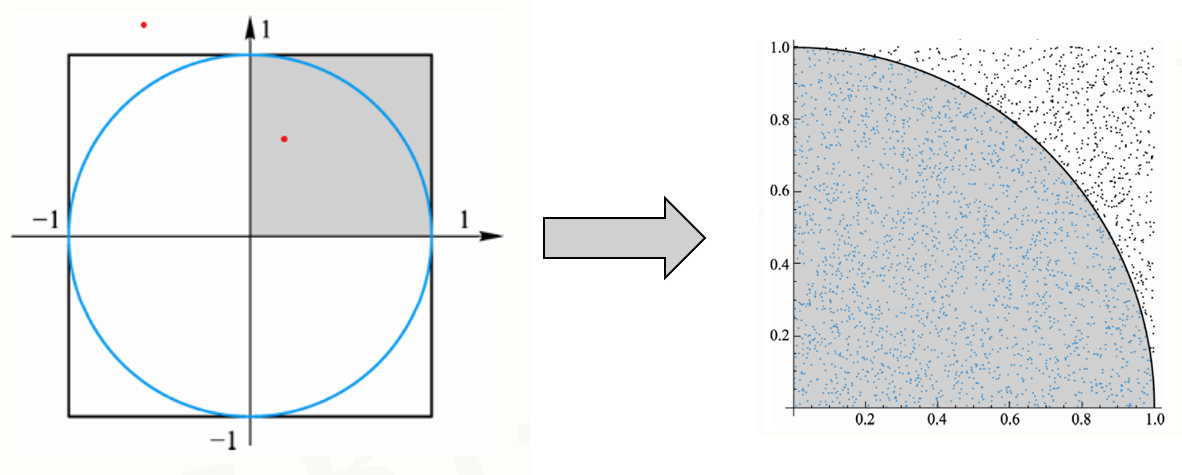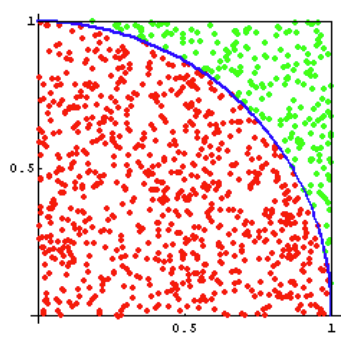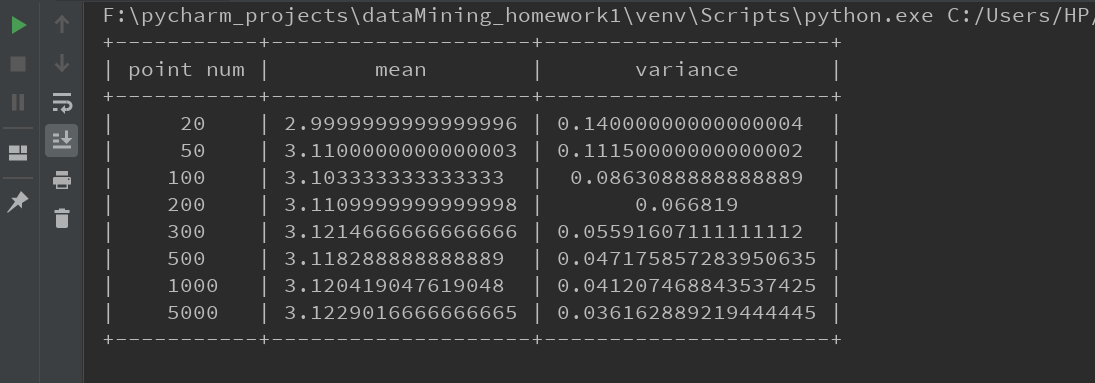• 蒙特卡洛方法求解圆周率的基本思路是对一个区域撒点，点的掉落是随机的，进行多次撒点后，计算点落在所区域内外的数量比得到所数值的近似值。 这个方法是计算思维的一个重要体现，即抽象一种过程，用计算机自动...
蒙特卡洛方法
蒙特卡洛方法求解圆周率的基本思路是对一个区域撒点，点的掉落是随机的，进行多次撒点后，计算点落在所求区域内外的数量比得到所求数值的近似值。
这个方法是计算思维的一个重要体现，即抽象一种过程，用计算机自动化求解。与依靠具体公式求解的数学思维相对立。
这里选取一个半径为1的圆的1/4，同时给出一个与之相切的正方形的1/4，利用这一部分进行重复的抛点试验。Python实现代码
from random import random #引入随机数函数
n = 1000*1000 #撒点的数量
hits = 0.0 #统计落在圆里的点的数量
for i in range(1,n+1):
x,y = random(),random() #随机抛点
dist =  pow(x**2+y**2,0.5) #随机点到圆心的距离
if dist<=1.0:
hits = hits + 1 #落在圆内部的点的数量
pi = 4*(hits/n) #圆内外点的对比
print("圆周率值是：{}".format(pi))

matlab实现代码
m = 0; %用来记录在圆的内部的点的个数
n = 10000;%试验次数
%假设圆的半径为1，也就是正方形的边长是2
for i = 1:n
x = rand;
y = rand;
if (x^2 + y^2) < 1   %如果点到圆形的距离小于半径1
m = m + 1;   %满足条件，记录次数加1
end
end
fprintf('圆周率值是：%d',4 * m/n);   %四倍的概率就是pi



展开全文• 蒙特卡洛方法求解圆周率pi 工具 python3.7 + pycharm 求解思路 首先使用random函数随机生成单位正方形之间的点，记录其分布情况，再出1/4圆内的点与单位正方形内点的比值，将该值乘以4即为pi值，重复上述...
蒙特卡洛方法求解圆周率pi工具

python3.7 + pycharm

求解思路

首先使用random函数随机生成单位正方形之间的点，记录其分布情况，再求出1/4圆内的点与单位正方形内点的比值，将该值乘以4即为pi值，重复上述过程20次，利用numpy.mean函数和numpy.var函数求出均值mean和方差variance。改变投点个数，记录对应的mean和variance，用PrettyTable绘制结果表格。

python源代码

1.	import random
2.	import numpy as np
3.	from prettytable import PrettyTable
4.
5.
6.	def getPi():
7.	    arr = []
8.	    table = PrettyTable(["point num", "mean", "variance"])
9.	    time = [20, 50, 100, 200, 300, 500, 1000, 5000]
10.	    for t in time:
11.	        # print(t)
12.	        for j in range(20):
13.	            cnt = 0
14.	            for i in range(t):
15.	                x = random.uniform(0, 1)  # 从一个均匀分布中随机采样，区间为左闭右开
16.	                y = random.uniform(0, 1)
17.
18.	                if (x * x + y * y) < 1:
19.	                    cnt += 1
20.	                    # 点分布在圆内的数量,因为正方形的面积为1，所以这个同时也是圆内的点与正方形内点的比值
21.	            vpi = 4.0 * (cnt / t)  # 4 * pi/4 = pi
22.	            arr.append(vpi)
23.	        mean = np.mean(arr)
24.	        variance = np.var(arr)
26.	    print(table)
27.
28.
29.	getPi()



运行结果展开全文机器学习
• 蒙特·卡罗方法（Monte Carlo method），也称统计模拟方法，是二十世纪四十年代中期由于科学技术的发展和电子计算机的发明，而被提出的一种以概率统计理论为指导的一类非常重要的数值计算方法。是指使用随机数（或更...
蒙特·卡罗方法（Monte Carlo method），也称统计模拟方法，是二十世纪四十年代中期由于科学技术的发展和电子计算机的发明，而被提出的一种以概率统计理论为指导的一类非常重要的数值计算方法。是指使用随机数（或更常见的伪随机数）来解决很多计算问题的方法。与它对应的是确定性算法。蒙特·卡罗方法在金融工程学，宏观经济学，计算物理学（如粒子输运计算、量子热力学计算、空气动力学计算）等领域应用广泛。
下面我们使用蒙特卡洛方法计算圆周率：
具体是，首先在一个x,y坐标系内，划分一个实际大小的正方形，然后假定向这个正方形里面洒黄豆，然后我们根据这个正方形的某一个点为圆心，画一个四分之一圆，进而利用数学方法，计算落在这个扇形里面的黄豆数量，和正方形里面的黄豆数量，然后根据数学关系即可计算出圆周率的值。自然地，这个正方形越大，往里面投的黄豆数量越多，越接近于实际的圆周率.
我们用计算思维来理解这件事，洒黄豆的行为我们可以借助于随机数来实现，然后我们给出代码：
#实例六：蒙特卡罗圆周率计算
from random import random
from time import perf_counter
darts = 1000 * 1000 #虚拟一个1000*1000的正方形
hit = 0.0
start = perf_counter()
for i in range(1,darts+1):
x,y = random(),random()
dist = pow(x**2+y**2,0.5)#计算点x,y到圆心的距离
if dist <= 1.0:
hit = hit +1
pi = 4 * (hit/darts)
print("圆周率是：{}".format(pi))
end = perf_counter() - start
print("运行时间是：{:.2f}S".format(end))


这里，我们假定这个正方形的规格是1000*1000，我们可以让这个面积更大，这样我们计算出的圆周率也就越准确


展开全文• 初学python，以概率的方法——蒙特卡洛方法求圆周率，以此练手
• import random k=0 sum=0 n=int(input('请输入要做的实验次数：')) for i in range(n): x,y=random.uniform(-1,1),random.uniform(-1,1) if x**2+y**2<...print('经过%d次实验之后所得圆周率的值为%f'%(n,pi))python
• 是按抽样调查法取统计值来推定未知特性量的计算方法。 代码实现 import numpy as np import matplotlib.pyplot as plt plt.rc('font', size=16) N = 10000 x, y = np.random.uniform(-1, 1, size=(2, N)) inside ...
蒙特卡罗法也称统计模拟法、统计试验法。是把概率现象作为研究对象的数值模拟方法。是按抽样调查法求取统计值来推定未知特性量的计算方法。
代码实现
import numpy as np
import matplotlib.pyplot as plt

N = 10000
plt.rc('font', size=16)
x, y = np.random.uniform(-1, 1, size=(2, N))
circle_in = x ** 2 + y ** 2 <= 1
circle_out = np.invert(circle_in)
pi = circle_in.sum() / N * 4
error = abs(np.pi - pi)/np.pi
plt.plot(x[circle_in],y[circle_in],'b.')
plt.plot(x[circle_out],y[circle_out],'r.')
plt.plot(0, 0, label='$\hat\pi$={:.4f}\nerror={:.4f}%'.format(pi, error), alpha=0)
plt.legend()
plt.axis('square')
plt.show()

结果图N数值越大，圆周率越准确，误差越小。


展开全文• Python 利用turtle库使用蒙特卡洛方法计算圆周率图片实例 from random import random import turtle as t from math import sqrt darts = 10000 hits = 0 t.penup() t.speed(0) t.tracer(False) for i in range(1,...
• 模拟大量随机计算，根据面积圆周率。 由概率比例 = 面积比例 可得出 pi 的计算公式 代码如下： #coding:utf-8 #python3 from random import random from time import perf_counter counts = 3000*3000...习题
• 初学python,今天尝试用 蒙特卡洛方法 算一下圆周率 蒙特卡方法: 蒙特卡洛方法是利用随机试验求解的问题。 如上图,在正方形内随机撒点，我们用园内点的数量除以整个点的数量就是面积的比，即四分之π。 代码...
• #蒙特卡洛方法计算圆周率 from random import random s=1000*1000#撒点的个数 hist=0.0#圆内的点的初值 for i in range(0,s+1): x=random() y=random() if pow(x**2+y**2,0.5)&lt;=1: hist+=1...
• [kou@hodge python]\$ cat circle.py #!/usr/bin/env python coding=utf-8 import time import random hits=0 pi=0 DARTS=100000*100000 start=time.perf_counter() for i in range(DARTS): x,y=random.random...
• #蒙特卡洛方法求解圆周率 from random import random from time import perf_counter DARTS = 1000*1000*10 hit = 0 star = perf_counter() for i in range(1,DARTS+1): x,y=random(),random() dist = pow(x ** 2+...
• 1.什么是蒙特卡洛方法(Monte Carlo method) 蒙特卡罗方法也称统计模拟方法，是1940年代中期由于科学技术的发展和电子计算机的发明，而提出的一种以概率统计理论为指导的数值计算方法。是指使用随机数（或更常见的伪...
• 这个大作业做的比较简单，其他同学动辄两三百行，我只是出于纯个人兴趣，想了解一下第三方库matplotlib的用法以及更加直观的了解蒙特卡洛方法可视化
• 一、蒙特卡洛法简介 ...二、蒙特卡洛法计算圆周率的原理 正方形的面积为： 圆的面积为： 圆的面积比上正方形的面积为：π / 4 所以我们使用蒙特卡洛法在正方形内随机撒点，落在圆内的点 / 落在正方形内...Java c语言
• 蒙特·卡罗方法（Monte Carlo method），也称统计模拟方法，是二十世纪四十年代中期由于科学技术的发展和电子计算机的发明，而被提出的一种以概率统计理论为指导的一类非常重要的数值计算方法。是指使用随机数（或更...
• 本文写给那些python初学者与对蒙特卡洛算法感兴趣，但却不知该如何理解或应用的人。（虽然我发现这个貌似有许多人做过了，但是程序都相对复杂，不便于理解，于是我就自己编写了一段程序，海龟的可视化请看下集如果...
• #阶乘函数 def myreduce(m): value = 1 for i in range(1,m+1): value = value*i return value #求和项的函数 def sumfun(m): div = (396**(4*m))*((myreduce(m))**4)#公式的分母部分 return (26390*m + ......

# 蒙特卡洛方法求圆周率pythonpython 订阅